Question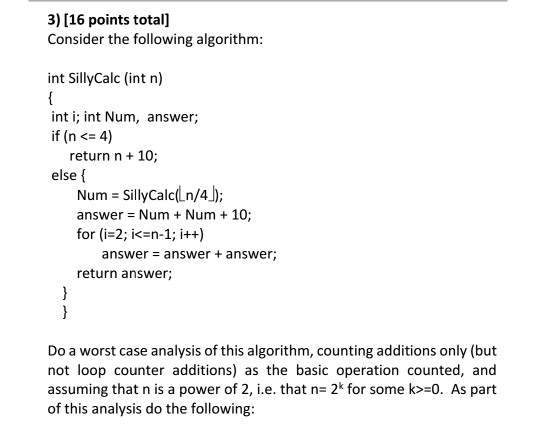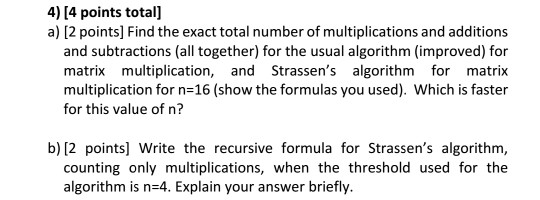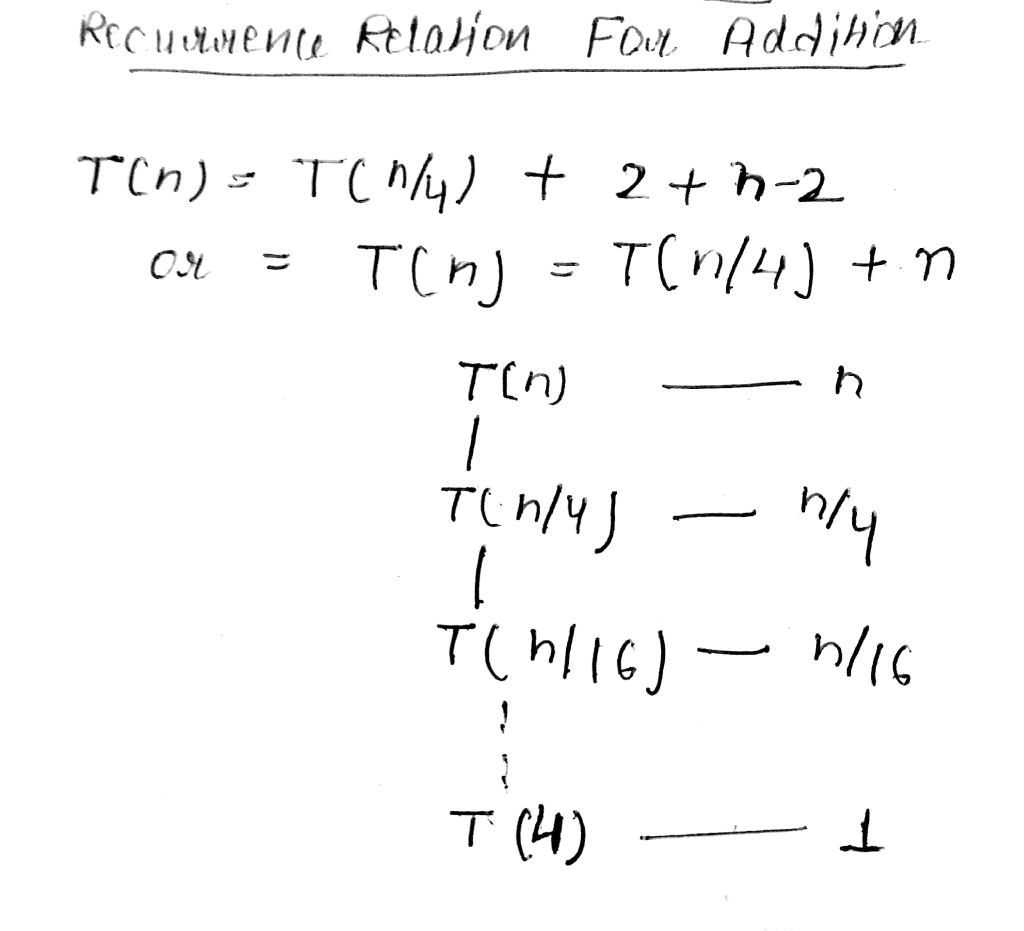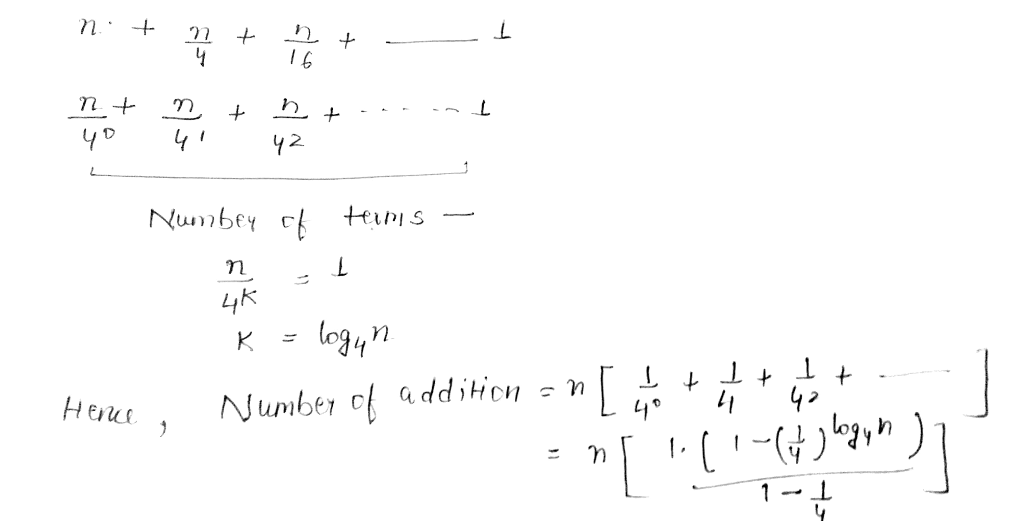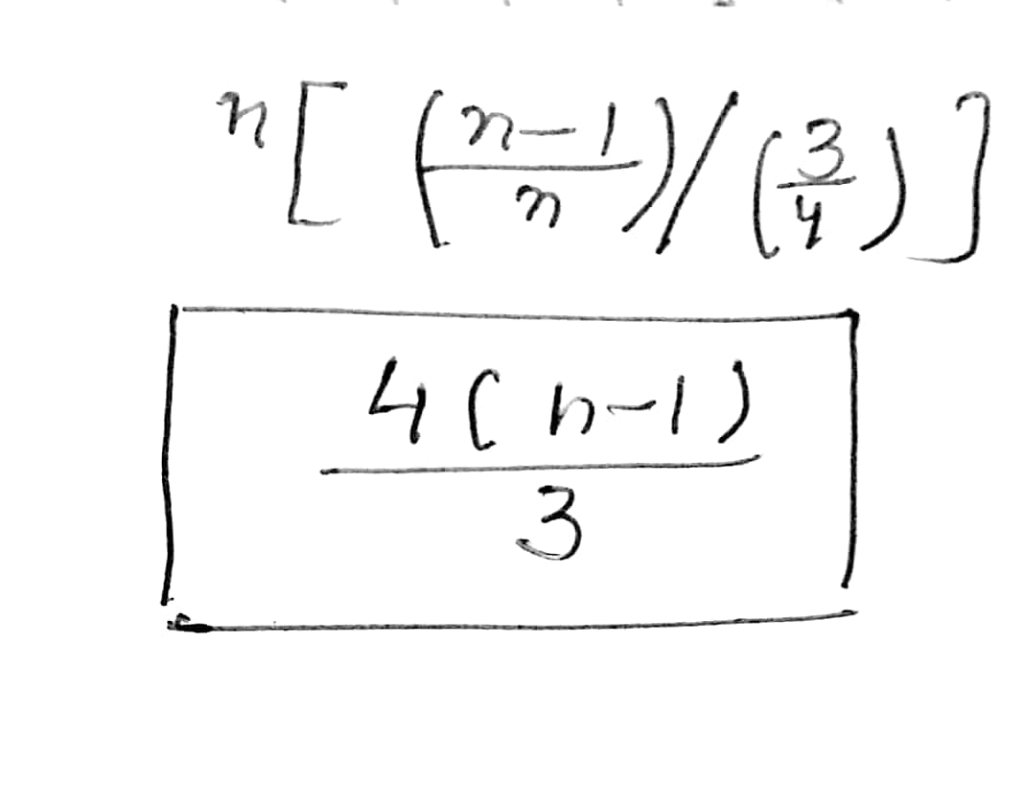#### Earn Coins

Coins can be redeemed for fabulous gifts.

Similar Homework Help Questions
• ### 3) [16 points total] Consider the following algorithm int SillyCalc (int n) int i; int Num, answer; if (n <=...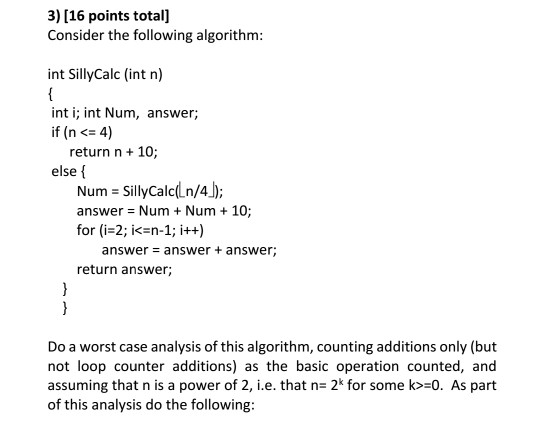3) [16 points total] Consider the following algorithm int SillyCalc (int n) int i; int Num, answer; if (n <= 4) return n 10; else { Num-SillyCalcl n/4) answer = Num + Num + 10; for (i-2; i<-n-1; ++) answer- answer+ answer; return answer; Do a worst case analysis of this algorithm, counting additions only (but not loop counter additions) as the basic operation counted, and assuming that n is a power of 2, i.e. that n- 2* for some...

• ### 3. Recursive Program (6 points) Consider the following recursive function for n 1: Algorithm 1 int...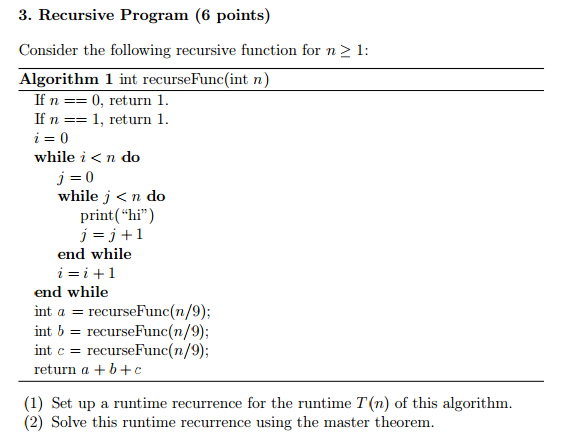3. Recursive Program (6 points) Consider the following recursive function for n 1: Algorithm 1 int recurseFunc(int n) If n 0, return 1. If n 1, return 1 while i< n do while j <n do print("hi") j 1 end while i i 1 end while int a recurse Func(n/9); int b recurse Func (n/9) int c recurse Func (n/9) return a b c (1) Set up a runtime recurrence for the runtime T n) of this algorithm. (2) Solve...

• ### 4. [16 marks total (6 marks each)] Do a worst-case analysis for the following algorithm segments, counting the number of multiplications which occur. I have marked the lines with the multiplications...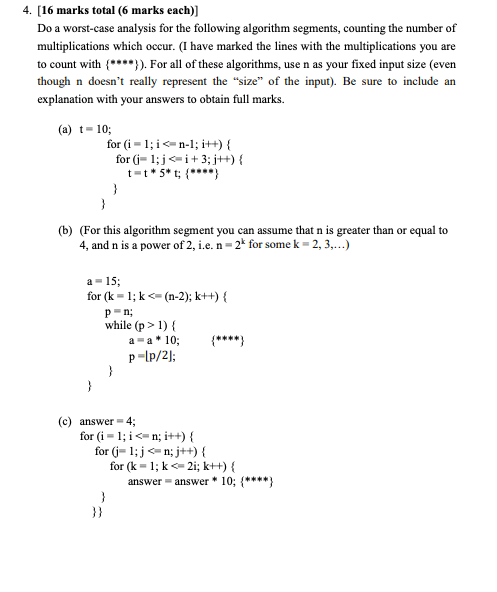4. [16 marks total (6 marks each)] Do a worst-case analysis for the following algorithm segments, counting the number of multiplications which occur. I have marked the lines with the multiplications you are to count with ). For all of these algorithms, use n as your fixed input size (even though n doesn't really represent the "size" of the input). Be sure to include an explanation with your answers to obtain full marks. (a) t-10; for (i-1;in-H) t-5*t; (b) (For...

• ### 4) [15 points total (5 points each)] Assume you are given a sorted array A of n numbers, where A is indexed from 1 up t...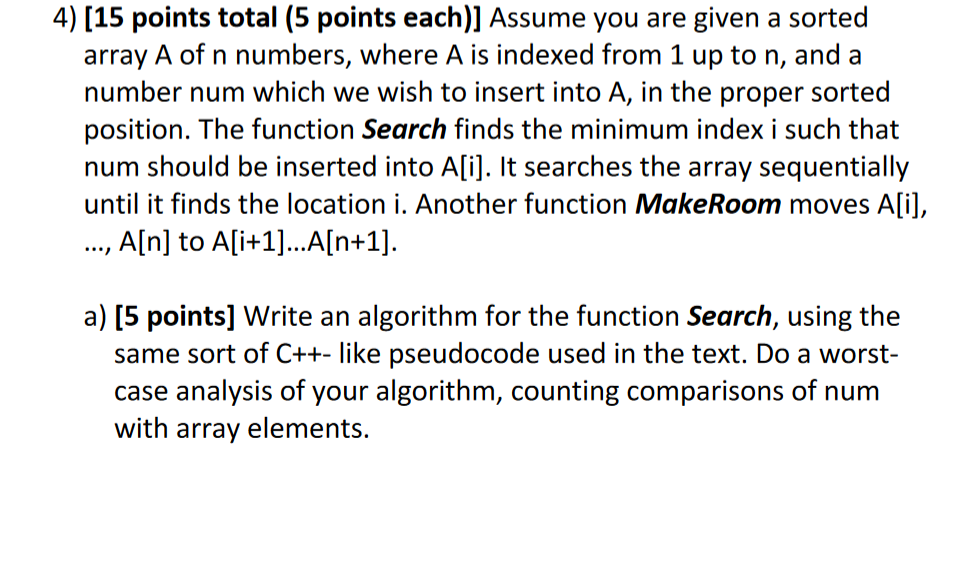4) [15 points total (5 points each)] Assume you are given a sorted array A of n numbers, where A is indexed from 1 up to n, anda number num which we wish to insert into A, in the proper sorted position. The function Search finds the minimum index i such that num should be inserted into Ali]. It searches the array sequentially until it finds the location i. Another function MakeRoom moves A[i], .., AIn] to Ali+1]...AIn+1] same sort...

• ### 2. Consider the function george (int n) computed by the following recursive C++ code. int george ...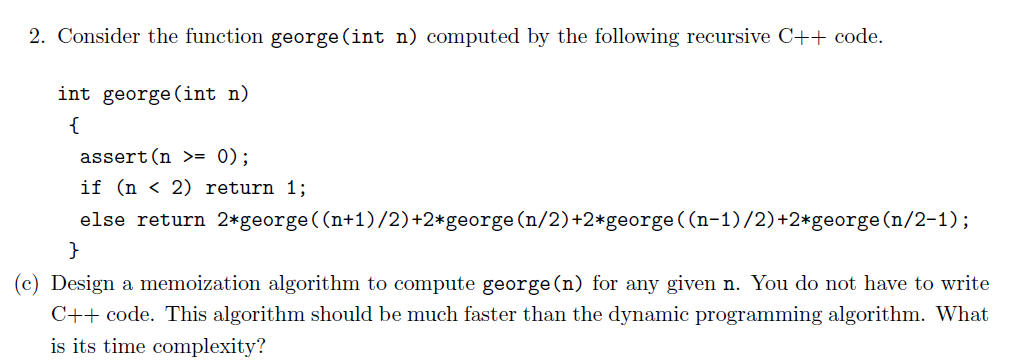2. Consider the function george (int n) computed by the following recursive C++ code. int george (int n) assert (n >= 0) if (n < 2) return 1; else return 2*george ((n+1)/2)+2*george (n/2)+2 george((n-1)/2)+2*george (n/2-1); (c) Design a memoization algorithm to compute george(n) for any given n. You do not have to write C++ code. This algorithm should be much faster than the dynamic programming algorithm. What is its time complexity? 2. Consider the function george (int n) computed by...

• ### Q5 (25pts) Consider the code: int foo(int N){ if (N <= 3) return 2; int res1 = 3*foo(N-4); int...

Q5 (25pts) Consider the code: int foo(int N){ if (N <= 3) return 2; int res1 = 3*foo(N-4); int res2 = foo(N-2); return res1-res2; } a) (6 points) Write the recurrence formula for the time complexity of this function (including the base cases) for N>=0. You do NOT need to solve it. b) (5 points) Draw the tree that shows the function calls performed in order to compute foo(8) (the root will be foo(8) and it will have a child...

• ### pleas answer asap 3. (20 points) Algorithm Analysis and Recurrence There is a mystery function called Mystery(n) and the pseudocode of the algorithm own as below. Assume that n 3* for some positiv...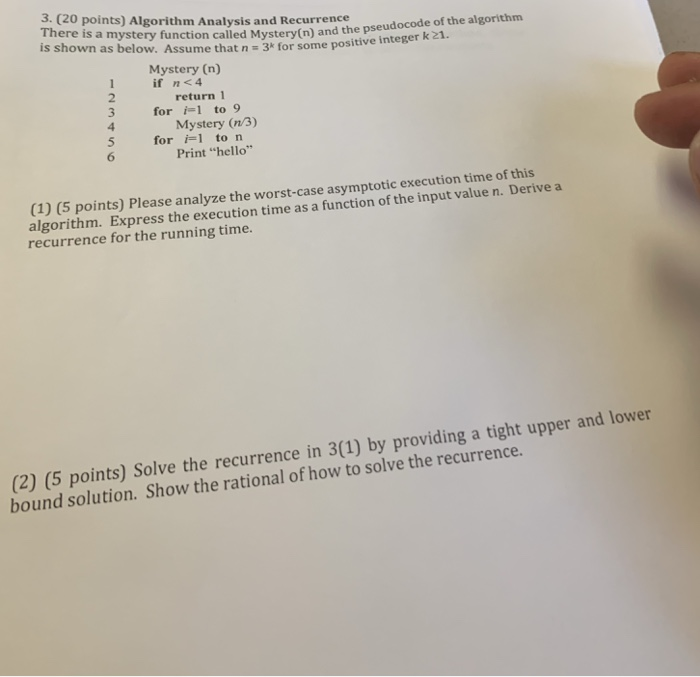pleas answer asap 3. (20 points) Algorithm Analysis and Recurrence There is a mystery function called Mystery(n) and the pseudocode of the algorithm own as below. Assume that n 3* for some positive integer k21. Mystery (n) if n<4 3 for i1 to 9 5 for i-1 to n 2 return 1 Mystery (n/3) Print "hello" 6 (1) (5 points) Please analyze the worst-case asymptotic execution time of this algorithm. Express the execution time as a function of the input...

• ### I already solved part A and I just need help with part B 1. Matrix Multiplication...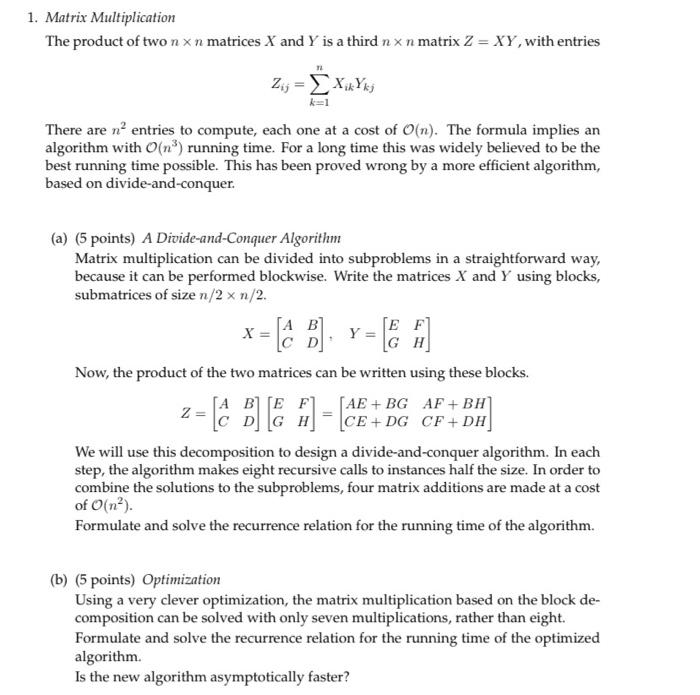I already solved part A and I just need help with part B 1. Matrix Multiplication The product of two n xn matrices X and Y is a third n x n matrix 2 = XY, with entries 2 - 21; = xixYk x k=1 There are n’ entries to compute, each one at a cost of O(n). The formula implies an algorithm with O(nº) running time. For a long time this was widely believed to be the best running...

• ### Consider the following function bubbleSort. int bubbleSort(int num[], int numel) {       int i, j, temp,...

Consider the following function bubbleSort. int bubbleSort(int num[], int numel) {       int i, j, temp, moves = 0;       for (i = 0; i < (numel - 1); i++)       {             for (j = 1; j < numel; j++)             {                   //missing code             }       } } A. Complete the missing code:- (4 Points) B. Assume that the array sort[] which has 8 elements, initially contains the values:- 22 5 67 98 45 32 101 99...

• ### please also explain how the answer came about if possible b) Base 7. Master Theorem (3...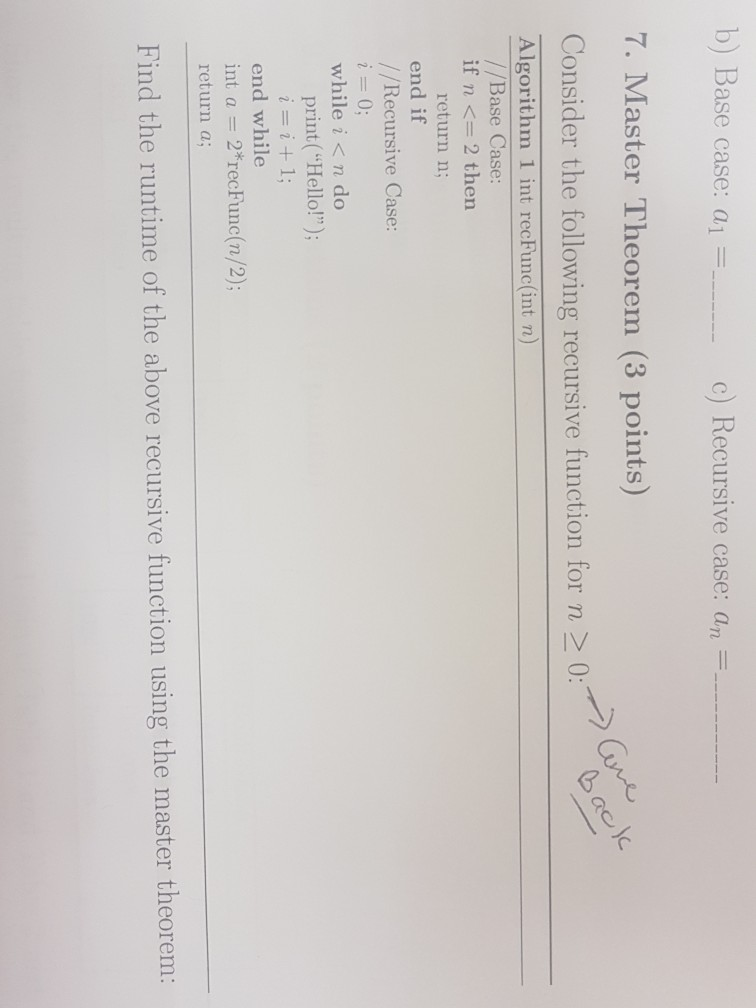please also explain how the answer came about if possible b) Base 7. Master Theorem (3 points) Consider the following recursive function for n >0 case: a| c) Recursive case: an Algorithm 1 int recFunc(int n) //Base Case if n <= 2 then return n; end if / /Recursive Case: while i< n do print("Hello!") end while int a 2*recFunc(n/2); return a; Find the runtime of the above recursive function using the master theorem